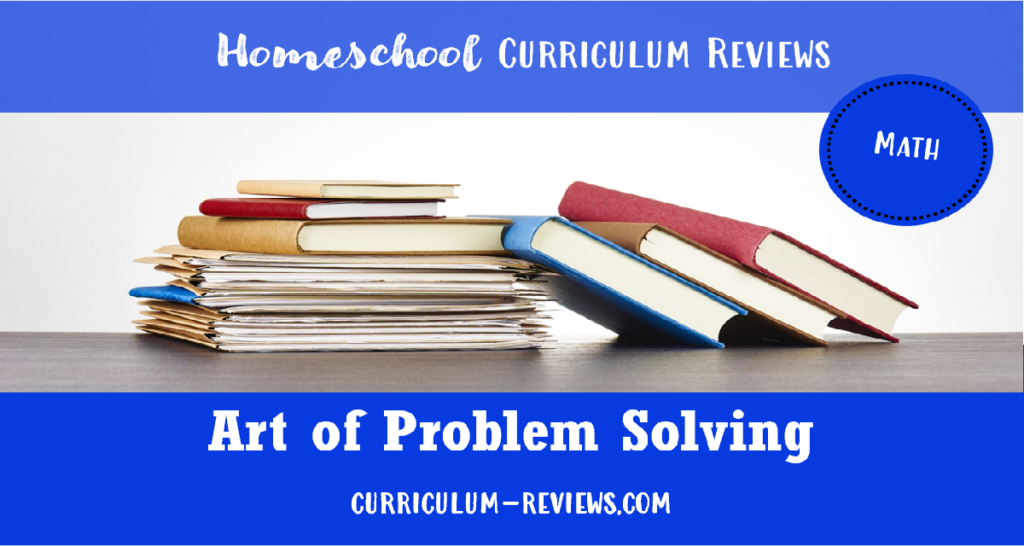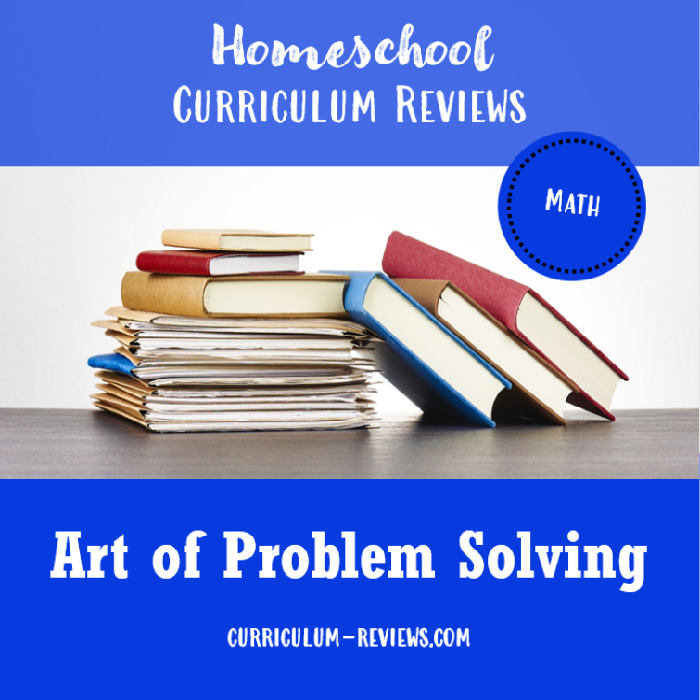Math### Art of Problem Solving, High School

Title: Art of Problem Solving, High School
Category:
Cost:
Tags:
Page Count: Varies
Format: Print
Published: 2011
Parent Involvement:

We’re just going to say it like it is: Art of Problem Solving is a math curriculum designed for kids who LOVE math and are really, really good at it. We’re talking about the kiddos who may be starting Algebra 1 at the age of 10.

That’s not to say that other students would not benefit from AoPS. But, if your teen enjoys math textbooks with the visual appeal of lots of bright pictures and sideboxes filled full of random fun facts and real world applications of math skills, then AoPS will probably not be a good fit.

AoPS textbooks are highly regarded for their rigor and depth, but they are dense and intense – which is not a bad thing, if you love math. Written in a conversational style, it’s like reading the transcript from a math lecture. Concepts are introduced and sample problems are explained step-by-step in text and number format.

Students then get the chance to try problems on their own: Exercise problems, review problems, and challenge problems. Don’t worry, though. The Solutions Manual not only gives you the answer, but it also gives you explanations on how to solve the harder problems.

Not sure what level you should start with? Visit the AoPS website’s Recommendation page and look for the Are You Ready? link to find a placement test for each textbook in the series.

Also, be sure to check out the Free Alcumus site on AoPS. You’ll find thousands of problems to supplement some of the textbooks. And, you can even watch video lessons that go with the Introduction to Counting & Probability book.

1. Introduction to Counting & Probability
Casework
Multiplication
Permutations
Combinations
Pascal’s triangle
Probability
Combinatorial identities
Binomial Theorem

2. Introduction to Geometry
Similar triangles
Congruent triangles
Polygons
Circles
Funky areas
Power of a point
Three-dimensional geometry
Transformations
and much more

3. Introduction to Number Theory
Primes and composites
Divisors and multiples
Prime factorization
Divisibility rules
Remainders
Modular arithmetic
Number bases
Linear congruences
How to develop number sense
and more

4. Intermediate Algebra
Review of basic algebra topics
Complex numbers
Polynomials
Multivariable expressions
Sequences and series
Identities
Inequalities
Exponents and logarithms
Piecewise-defined functions
Functional equations
and more

5. Intermediate Counting & Probability
Inclusion-exclusion
1-1 correspondences
Pigeonhole Principle
Constructive expectation
Fibonacci and Catalan numbers
Recursion
Conditional probability
Generating functions
Graph theory
and more

6. Pre-Calculus
Trigonometry
Complex numbers
Vectors
Matrices

7. Calculus
Limits
Continuity
Derivatives
Integrals
Power series
Plane curves
Differential equationsAbout the Authors: Richard Rusczyk is the founder of the Art of Problem Solving website. He was a national MATHCOUNTS participant in 1985, a three-time participant in the Math Olympiad Summer Program, a perfect AIME scorer in 1989, and a USA Mathematical Olympiad winner. He is author or co-author of 6 Art of Problem Solving textbooks. Sandor Lehoczky participated in the Math Olympiad Summer Program in 1989, and in 1990 earned the sole perfect AIME score and led the national first place team on the AHSME (now AMC 12). Lehoczky and Rusczyk were co-founders of the Mandelbrot Competition.